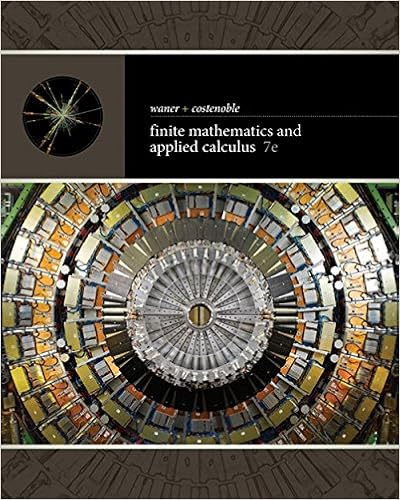# In the preceding diagram what areas represent

• 4
• 100% (2) 2 out of 2 people found this document helpful

This preview shows page 2 - 4 out of 4 pages.

##### We have textbook solutions for you!
The document you are viewing contains questions related to this textbook.The document you are viewing contains questions related to this textbook.
Chapter 12 / Exercise 7
Finite Mathematics and Applied Calculus
Costenoble/WanerExpert Verified
2. 2. In the preceding diagram, what areas represent consumers’ surplus at the equilibrium price of P E ? At P C ? (Keep in mind that, at P C , the equilibrium quantity is neither produced nor sold.)
##### We have textbook solutions for you!
The document you are viewing contains questions related to this textbook.The document you are viewing contains questions related to this textbook.
Chapter 12 / Exercise 7
Finite Mathematics and Applied Calculus
Costenoble/WanerExpert Verified
III. Chapter 6: Questions & Problems: 2, 5, 9, 14 (p175-176) Working with Numbers and Graphs: 5 (p176) 2. 2. For each of the following, identify where demand is elastic, inelastic, perfectly elastic, perfectly inelastic, or unit elastic: (a) price rises by 10 percent and the quantity demanded falls by 2 percent; (b) price falls by 5 percent and the quantity demanded rises by 4 percent; (c) price falls by 6 percent and the quantity demanded does not change; (d) price rises by 2 percent and the quantity demanded falls by 1 percent.
5. 5. Identify whether total revenue rises, falls, or remains constant for each of the following: (a) Demand is inelastic and price falls; (b) Demand is elastic and price rises; (c) Demand is unit elastic and price rises; (d) Demand is inelastic and price rises; (e) Demand is elastic and price falls.
9. 9. How might you determine whether toothpaste and mouthwash manufacturers are competitors?
•••